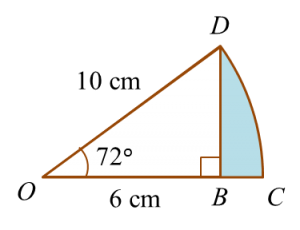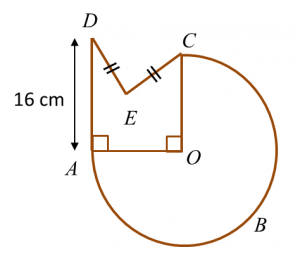10.2.2 Circles I, PT3 Focus Practice

Question 6:
In the diagram below, CD is an arc of a circle with centre O.Determine the area of the shaded region.

Solution:

Question 7:
In diagram below, ABC is a semicircle with centre O.Calculate the area, in cm2 , of the shaded region.

Solution:

Question 8:
In diagram below, ABC is an arc of a circle centre OThe radius of the circle is 14 cm and AD = 2 DE.
Calculate the perimeter, in cm, of the whole diagram.

Solution:

Question 9:
In diagram below, KLMN is a square and KLON is a quadrant of a circle with centre K.Calculate the area, in cm2, of the coloured region.

Solution:

Question 10:
Diagram below shows two quadrants, AOC and EOD with centre O.Sector AOB and sector BOC have the same area.
Calculate the area, in cm2, of the coloured region.

Solution: